# How can i round this number

You can write your topic however you want, but you need to answer these questions:

1. What do you want to achieve? Keep it simple and clear!
A: i want to round out some numbers in a gui so its easier to be read

2. What is the issue? Include screenshots / videos if possible!
A: i cant get the gui to round out these numbers in it, i tried using math.floor and math.ceil but nothing changed.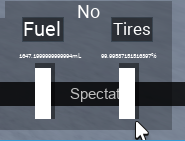(Sorry for the low quality, i work on a 1360x768 laptop screen.)

1. What solutions have you tried so far? Did you look for solutions on the Developer Hub?
A: as i said earlier, i tried using math.floor, math.ceil and other things i found on the devforum, but my gui always keeps saying, for example: .999999999999999999978 (as shown above in the screenshot, same with the tires)

here is the part of the code that measures the fuel.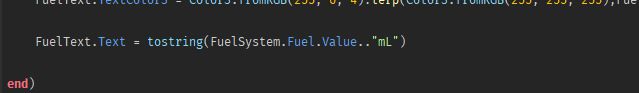and here is the one that updates the text.

im a little new to doing math in code, so i cant wrap my head around what i can do to fix this.
this is also my very first post so i apologize if i did anything wrong by posting this.

1 Like
``````local function round(n)
return math.floor(n + 0.5)
end
``````

I actually forgot `math.round()` exists, sorrythere’s always `math.round()` if you need it

1 Like

i also tried math.round but it didnt work either, i still get the same .99999999999 decimal with it

If you actually get only .9999999, then use `math.ceil()`

actually i just noticed something on the gui

the starter number of fuel is 1650 ml which each milisecond goes down by 0.2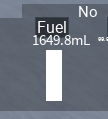but, when the number reaches below 1,694.6 it ends up saying 0.999999999999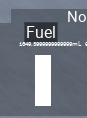I think it might be imprecision of calculation, which might be a studio bug. There should be a workaround.

tried it but now i get this error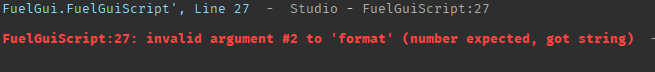i could be setting it wrong but im not very sure of it

i also tried putting it on the part of the code where it calculates the fuel, but it kept saying the 0.9999999999

This thread mentions the same issue. It might be unfixable, but I refuse to believe it, as there are plenty of games having similar mechanic to yours and they work fine.

What you want here is string.format. tostring will give you an exact representation of the number.

``````FuelText.Text = string.format("%.1f mL", FuelSystem.Fuel.Value)
``````

That `%.1f` part of that determines how it should round. `%d` would be no decimals. `%.3f` would be three decimals.

3 Likes

Actually I had the same problem 3-4 months ago and found this post with the solution.

``````local dec = 2

local function round_to_nearest_num(num, dec)
dec = dec or 1

local k = 10^dec
return math.round(num*k)/k
end

print(round_to_nearest_num(1693.99999999999999, dec)) -- 1694
print(round_to_nearest_num(0.6777777777777, dec)) -- 0.68
print(round_to_nearest_num(1.911111111, dec)) -- 1.91
``````

thank you so much, now that i notice i was putting the string.format wrong.